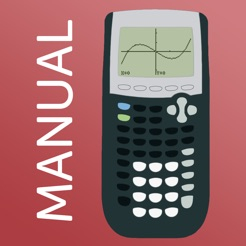## Screenshots

•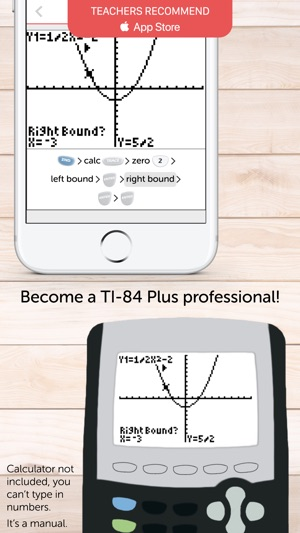•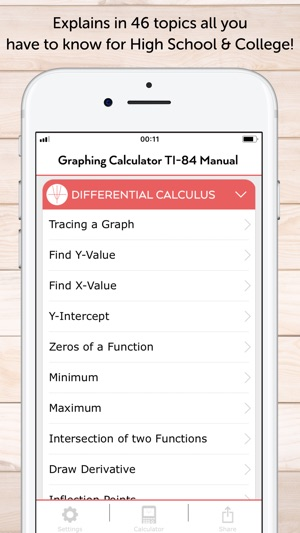•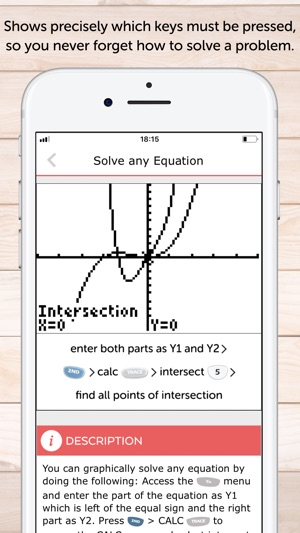•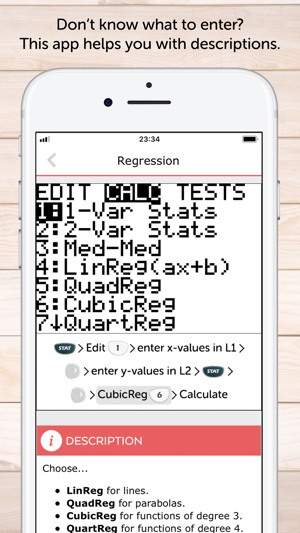•## Description

The TI-84 Graphing Calculator Manual app is ranked TOP 25 Education USA!

How this app makes math so much easier:
• This manual shows all important functions of the graphing calculator TI-84 Plus, which are very useful for high school and college. Actual calculator not included!
• Also suited for TI-83 Plus, TI-84 Plus Silver Edition, TI-84 Plus C.
• The app shows the exact key press sequence for 46 topics. It covers the chapter:s First Steps, Graphing Basics, Differential Calculus, Solve Equations, Integral Calculus, Matrices and Statistics & Probability.
• You can swipe through screenshots of the original graphing calculator which show every step you have to do (220 screenshots in total) or watch small animations to see how it works on the TI-84!
• Additionally, there are helpful descriptions of further knowledge and useful tips.
• More than 60 math problems with solutions to practice your skills!

Not yet convinced? Here's how you would benefit from this app:
• Every topic shows a detailed instruction through screenshots of the original graphing calculator TI-84 Plus which explain how to use the calculator. You only have to swipe right to get the next screenshot which looks similar to your calculator display!
• The app guides you with easy to understand explanations and offers the ability to show animations. It's like your virtual teacher.

• The app is also ideal for exam preparation as you often don't remember all instructions of the calculator after several months. It covers all you need to know for the ACT and SAT.
• Your teacher will be grateful as well because he doesn't have to walk around the classroom until everyone's calculator shows the right solution. You only have to take a look at the app and know how to do!

• The app contains the following topics:

FIRST STEPS
• The Basics of the Basics
• Mode Settings
• Basic Arithmetic
• Storing Variables
• Working with Fractions
• Testing Numbers
• Converting Angles & DMS

GRAPHING BASICS
• Enter Functions
• Enter Families of Functions
• Formatting the Graph
• Setting the Graph Window
• Zooming the Graph Window

DIFFERENTIAL CALCULUS
• Tracing a Graph
• Find Y-Value
• Find X-Value
• Y-Intercept
• Zeros of a Function
• Minimum
• Maximum
• Intersection of two Functions
• Draw Derivative
• Inflection Points
• Calculate Tangent

SOLVE EQUATIONS
• Polynomial
• Solve any Equation
• Equation Solver

INTEGRAL CALCULUS
• Calculate Integral
• Integral in GRAPH-Menu
• Find Area with Absolute Value
• Area between two Functions
• Integral Function

MATRICES
• Save Matrix
• Delete Matrix
• Put into Row Echelon Form
• Solve Matrix
• Determinant of a Matrix
• Transpose of a Matrix
• Identity Matrix
• Inversion of a Matrix
• Matrix Arithmetic Operations

STATISTICS & PROBABILITY
• Permutations, Combinations & Factorials
• Random Numbers
• Lists & Statistical Data
• Histogram, Box Plot & Scatter Plot
• Statistical Data Analysis
• Regression

MATH PROBLEMS
• Practice with over 60 math problems and solutions.
• You can practice math problems of a single topic (e.g., Zeros of a Function) or random math problems of Differential Calculus for example.
• As an alternative, the app shows you mixed math problems of all topics.

We also have apps for the calculators: TI-Nspire CX (and CAS), TI-84 Plus, TI-84 Plus CE, CASIO fx-9860GlI

NO ACTUAL GRAPHING CALCULATOR INCLUDED. THE APP IS A MANUAL!

## What’s New

Version 1.5.6

+ Updates to the descriptions of most TI 84 calculator chapters
+ Performance improvements & Bug Fixes​

## Ratings and Reviews

3.2 out of 5
134 Ratings
Lashell Clack ,

### Works very well, very easy to use

I used many other applications but this is an actual app that I was seeking for. It covers all the important concepts which help me making my graphing calculations easy.

joshv.91.11.1 ,

### TI 84 calculator manual

This manual for the TI-84 graphing calculator is awesome! It helped me so much to prepare for tests and the ACT. It teaches you everything you must know about the graphing calculator and is easy to understand. You should really get this if you have a TI 84 grapher!

Mavis Stucker ,

### Thanks for developer!!

I love all the features of this app like graphing basic, differential calculus, integral calculus & math problem. After using this app my interest level in Math’s get hike.

## Information

Seller
Graphing Calculator Apps UG (haftungsbeschrankt)
Size
42.5 MB
Category
Education
Compatibility

Requires iOS 9.0 or later. Compatible with iPhone, iPad, and iPod touch.

Languages

English

Age Rating
Rated 4+
© 2018 Economic Solution Apps
Price
\$4.99

## Supports

•